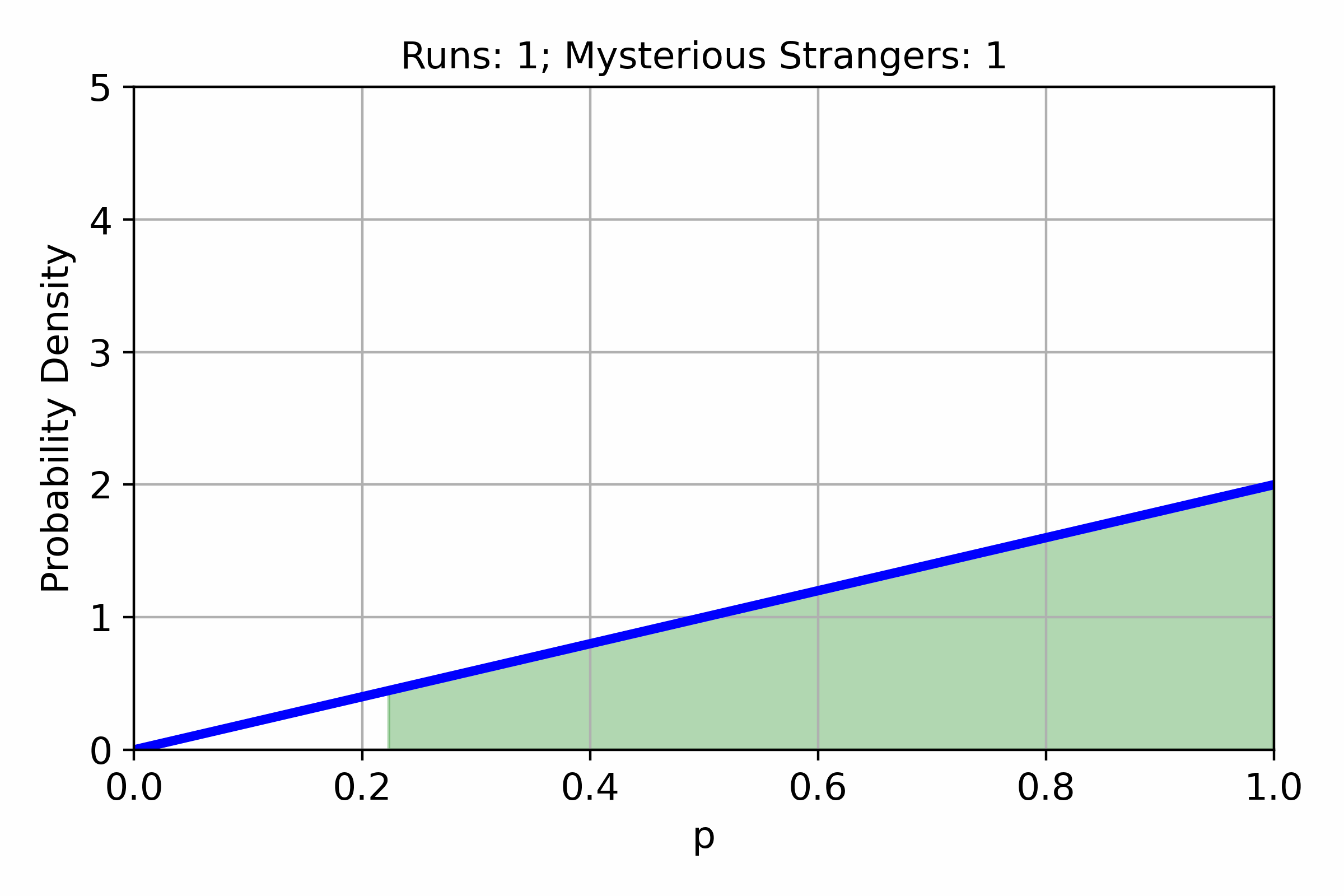Estimating drop chances with Bayesian statistics

In many games, different items or events have an associated chance to appear. Often these chances are unknown to the players. In this post, we will try to estimate the chance of getting a Mysterious Stranger in Hearthstone Mercenaries from...In Hearthstone battlegrounds as of patch 18.6.1 the Amalgadon card have the following effect: Battlecry: For each different minion type you have among other minions, Adapt randomly. When playing Amalgadon the number of adapts will be in the range between...Overlap between Danish investment funds

Given the selection of Danish investment funds from Sparindex and Danske Invest, it can be difficult to see how different the funds are. As a measure of similarity, one can calculate the overlap between two funds. The overlap between fund...Model for the price of Danish investment funds

In Denmark, all ETFs are taxed yearly by unrealized returns. On the other hand, some Danish investment funds are taxed by realized gain, if they fulfill some criteria of paid out dividends. The dividend paid out will be: earned dividends...Brokerage vs. oppertunity cost

Many brokers (at least in Denmark), have a pricing model of $$q\%$$ of the trading amount or minimum $$q$$ Euro. Given a fixed amount to invest every month, we might think it is best to accumulate the cash to reach...Multipole fit with Lagrangian multipliers

Consider the electrostatic potential due to multipoles places at the position of atoms: $E_{i}=\sum_{j}^{atom}\sum_{n}^{multipole}\frac{\left(-1\right)^{n}}{n!}T_{ij}^{(n)}m_{j}^{(n)}$ If the quantum mechanical electrostatic potential is to be minimized, a cost function can written of the form: $z=\sum_{i}^{point}\left(V_{i,\mathrm{QM}}-E_{i}\right)^{2}+\sum_{l}^{constraints}\lambda_{l}g_{l}$ Expanding the square: $z=\sum_{i}^{point}\left(V_{i,\mathrm{QM}}^{2}+E_{i}^{2}-2E_{i}V_{i,\mathrm{QM}}\right)+\sum_{l}^{constraints}\lambda_{l}g_{l}$ It is known...

Second order partial derivative for multivariate function, derivation

Consider a function: $f\left(x_{1}\left(\lambda_{1},\lambda_{2},..,\lambda_{M}\right),x_{2}\left(\lambda_{1},\lambda_{2},..,\lambda_{M}\right),..,x_{N}\left(\lambda_{1},\lambda_{2},..,\lambda_{M}\right)\right)$ The first partial derivative is given as: $\frac{\partial}{\partial\lambda_{i}}f\left(x_{1},x_{2},..,x_{N}\right)=\frac{\partial f}{\partial x_{1}}\frac{\partial x_{1}}{\partial\lambda_{i}}+\frac{\partial f}{\partial x_{2}}\frac{\partial x_{2}}{\partial\lambda_{i}}+...+\frac{\partial f}{\partial x_{N}}\frac{\partial x_{N}}{\partial\lambda_{i}}$ The above equation can be formulated as: $\frac{\partial}{\partial\lambda_{i}}f\left(x_{1},x_{2},..,x_{N}\right)=\sum_{k}^{N}\frac{\partial f}{\partial x_{k}}\frac{\partial x_{k}}{\partial\lambda_{i}}$ Now consider the second derivative: \[\frac{\partial^{2}}{\partial\lambda_{j}\partial\lambda_{i}}f\left(x_{1},x_{2},..,x_{N}\right)=\frac{\partial}{\partial\lambda_{j}}\left(\frac{\partial f}{\partial x_{1}}\frac{\partial...